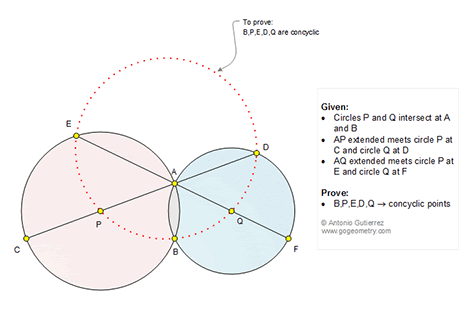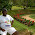## Tuesday, July 12, 2016

### Geometry Problem 1236: Intersecting Circles, Secant, Concyclic Points

Geometry Problem. Post your solution in the comment box below.
Level: Mathematics Education, High School, Honors Geometry, College.

This entry contributed by Sumith Peiris, Moratuwa, Sri Lanka.

Click the figure below to view more details of problem 1236.1.Isosceles triangles PEA and QDA are similar => EA/PA = DA/QA => EA.AQ = PA.AD
Hence E,P,Q and D are concyclic.

B also lies on the same circle

1."B also lies on the same circle"

Could u pls explain how u arrived at this conclusion?

2.<PAQ = <PBQ = 135 which are supplimentary. Hence B also lies on the same circle. For some reasons, this did not get printed in my first comment. Faced this trouble couple of times and I stopped posting. ( I have posted <PEA = <PEQ = 45 in the beginning, but the preview is truncating this as well)

3.< PAQ = < PBQ = 135. How did u arrive at this conclusion?

2.connect PB, BQ, <PBQ = <PAQ,
<PAQ + <PAE = 180 = <PAQ + <PEA =< PBQ + <PEA =180,
SO b ALSO LIES ON THE SAME CIRCLE

3.Isosceles triangles PEA and QDA. Since <PBQ+<E=<PAQ+<EAP=180, E,P,B,Q concyclic. Similary, D,Q,B,P concyclic. Therefore, E,P,B,Q,D concyclic.

4.Problem 1236
Is <EPA=2.<EBA (PE=PA), <AQD=2.<ABD (AQ=QD).But <EAP=<DAQ=x. Then <EPD=<EPA=
180-2x=2(90-x)=<AQD=<EQD.But <EBD=<EBA+<ABD=(< EPA)/2+(<AQD)/2=90-x+90-x=180-2x.Therefore points E,P,B,Q and D are concyclic.
APOSTOLIS MANOLOUDIS 4 HIGH SHCOOL OF KORYDALLOS PIRAEUS GREECE

5.The solution that I propose is the following:
<PEA=<ADQ=<EAQ=<DAQ=x ; <EPA=AQD=y= 180-2x
<PAB=<PBA=α ; <APB=K=180-2α
<QAB=<ABQ=β ;<AQB=R=180-2β ; <ABQ+<ABP = α + β
To be concyclic the points BPED and Q need that:
<R+<K+<y=180 or α + β +x= 180 ;
<R+<K+<y=180-2β+180-2α+180-2x=α + β +x=180
Therefore point BPED and Q are concyclic
6.